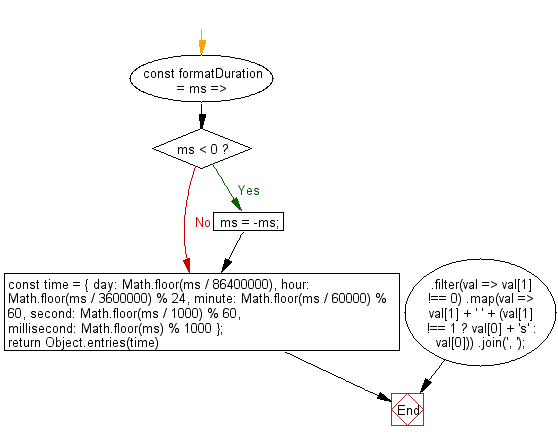# JavaScript: Get the human readable format of the given number of milliseconds

## JavaScript fundamental (ES6 Syntax): Exercise-230 with Solution

Write a JavaScript program to generate the human-readable format in the given number of milliseconds.

• Divide ms with the appropriate values to obtain the appropriate values for day, hour, minute, second and millisecond.
• Use Object.entries() with Array.prototype.filter() to keep only non-zero values.
• Use Array.prototype.map() to create the string for each value, pluralizing appropriately.
• Use String.prototype.join(', ') to combine the values into a string.

Sample Solution:

JavaScript Code:

``````//#Source https://bit.ly/2neWfJ2
const formatDuration = ms => {
if (ms < 0) ms = -ms;
const time = {
day: Math.floor(ms / 86400000),
hour: Math.floor(ms / 3600000) % 24,
minute: Math.floor(ms / 60000) % 60,
second: Math.floor(ms / 1000) % 60,
millisecond: Math.floor(ms) % 1000
};
return Object.entries(time)
.filter(val => val !== 0)
.map(val => val + ' ' + (val !== 1 ? val + 's' : val))
.join(', ');
};

console.log(formatDuration(1001));
console.log(formatDuration(34325055574));
```
```

Sample Output:

```1 second, 1 millisecond
397 days, 6 hours, 44 minutes, 15 seconds, 574 milliseconds
```

Flowchart:Live Demo:

See the Pen javascript-basic-exercise-230-1 by w3resource (@w3resource) on CodePen.

Improve this sample solution and post your code through Disqus

What is the difficulty level of this exercise?

Test your Programming skills with w3resource's quiz.

﻿